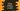# Write a python program to reverse a number# Python program to reverse a number :

This is one of the most common questions asked in a Junior level programming interview. I have not found any real project that requires to reverse a number. Maybe if you are implementing some kind of logic or puzzle games, this program will come in handy.

If you are learning to programme or starting to learn python, then I would highly recommend you to go through this blog post. Don’t just copy-paste the program. Read the post, minimize the browser and then try to solve it without googling.

We will learn how to_ reverse a number_ in python in this post. Our program will take one integer number as an input from the user, reverse it and print out the reverse number.

For example, if the number is 154, the program will print _451 _as the output.

Note that for a single digit number, the output will be the same. Also, this program works only with positive numbers.

## The algorithm to solve this problem :

Following are the steps we will use to solve the problem :

1. Take the number as input from the user.
2. Take the rightmost digit of the number and add it to the first place of the resulting number.
3. Remove the last digit.
4. Similarly, remove the last digit of the current modified number continuously and keep adding it to the end of the final number using a loop.
5. After the loop is completed, print out the final reverse number to the user.

## Python program :

The python program to solve the problem is as below :

``````#1
num = int(input("Enter a number: "))

#2
reverse_num = 0

#3
while(num>0):
#4
remainder = num % 10

#5
reverse_num = (reverse_num * 10) + remainder

#6
num = num//10

#7
print("The reverse number is : {}".format(reverse_num))``````

### Explanation :

The commented numbers in the above program denote the step numbers below :

1. Ask the user to enter a number. Read it and store it in the num variable.
2. Initialize one variable reversenum_ to store the final reversed number. Initialize it to 0.
3. Run one while loop. Run the loop until the value of num is greater than 0.
4. Find the remainder of the variable num and store it in the remainder variable.
5. Update the reversenum_. In this step, we are adding the remainder to its appropriate position.
6. Change the value of num to num//10. For example, it the number was 145, it will become 145/10 = 14. (Not 14.5 because num is an integer variable, so it can’t hold_ 14.5_ )
7. Finally, print out the reverse number reversenum_.

Note that the user given number num is modified in this program. If you want to print the user given number along with the modified number, keep it in a separate variable.

If you got confused, let me explain it with an example. Suppose, we are finding the reverse number of 453. The steps will be as below :

After step 3, the while loop will exit and the reversed number will be printed as 354.

### Conclusion :

In this tutorial, we have learned how to find the reverse of a number in python. This program will run only once, i.e. it will ask the user to enter a number, find out the reverse value, print it out and exit. We can also insert it inside an infinite loop to keep reading a new number from the user continuously. Put it inside an infinite loop and check what happens. If you have any queries or anything you want to add in this post, don’t hesitate to drop a comment below.